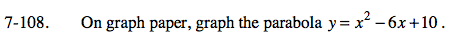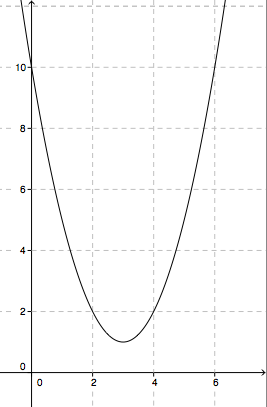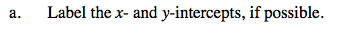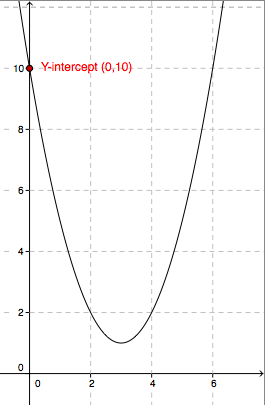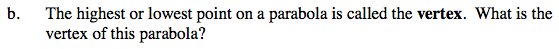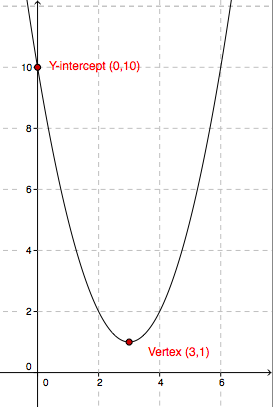Home > CAAC > Chapter 7 > Lesson 7.3.3 > Problem7-108

7-108.
1. On graph paper, graph the parabola y = x2 − 6x + 10. Homework Help ✎

1. Label the x- and y-intercepts, if possible.

2. The highest or lowest point on a parabola is called the vertex. What is the vertex of this parabola?